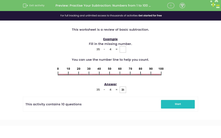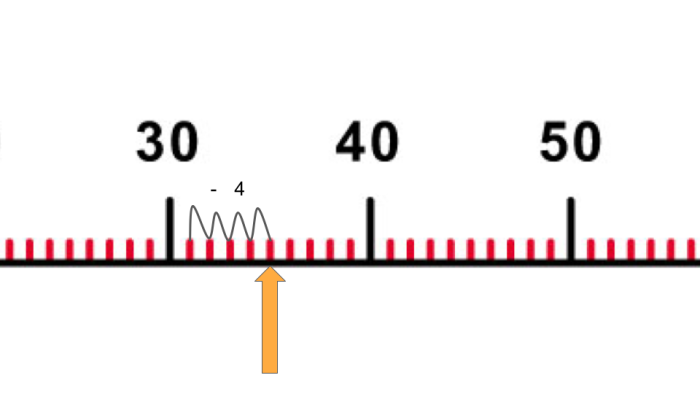# Practise Your Subtraction Skills with Numbers up to 100

In this worksheet, students will practise their subtraction skills.Key stage:  KS 1

Curriculum topic:   Number: Addition and Subtraction

Curriculum subtopic:   Use Addition/Subtraction Facts to 100

Difficulty level:#### Worksheet Overview

In this activity, we will be answering some simple subtraction questions.

Example

Fill in the missing number.

 35 − 4 =35 - 4 = 31

Tips

Remember, when the second number in the number sentence is missing, we can subtract the number after the equals sign to find the missing number.

 9 − = 3

To solve this we would do 9 - 3 = 6

When it is the first number missing, we add together the other two numbers to find the missing number.

 − 5 = 9

To solve this we would do 5 + 9 = 14

Are you happy with that?Let's get started.

### What is EdPlace?

We're your National Curriculum aligned online education content provider helping each child succeed in English, maths and science from year 1 to GCSE. With an EdPlace account you’ll be able to track and measure progress, helping each child achieve their best. We build confidence and attainment by personalising each child’s learning at a level that suits them.

Get started